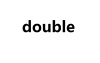double 订阅
double是一个英语单词，意为一对，一双；双重地；酷似的人；把...对折；变成两倍；增加一倍，可作形容词、副词、动词用。 展开全文double是一个英语单词，意为一对，一双；双重地；酷似的人；把...对折；变成两倍；增加一倍，可作形容词、副词、动词用。

double

double单词

• Double 基本数据类型double 的包装类 Double 类型的对象包含一个 double 类型的字段 属性简介 用来以二进制补码形式表示 double 值的比特位数 public static final int SIZE = 64; 二进制补码形式表示 ...
Double 基本数据类型double  的包装类

Double 类型的对象包含一个 double 类型的字段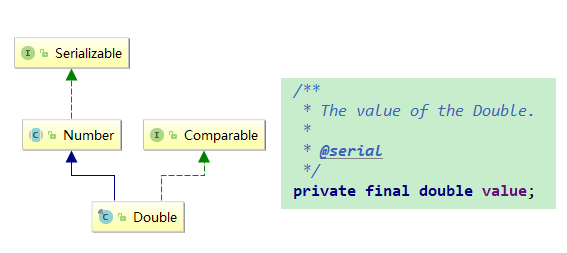属性简介

用来以二进制补码形式表示 double 值的比特位数
public static final int SIZE = 64;
二进制补码形式表示 double 值的字节数
public static final int BYTES = SIZE / Byte.SIZE;
表示基本类型 double 的 Class 实例
public static final Class<Double>   TYPE = (Class<Double>) Class.getPrimitiveClass("double");
能够表示的最大值
只有标准化一种形式,也就是前文提到过的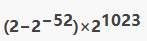public static final double MAX_VALUE = 0x1.fffffffffffffP+1023;
标准化的最小值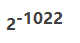public static final double MIN_NORMAL = 0x1.0p-1022;
最小值还有非标准化的形式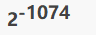public static final double MIN_VALUE = 0x0.0000000000001P-1022;
正无穷
等同于Double.longBitsToDouble(0x7ff0000000000000L)
public static final double POSITIVE_INFINITY = 1.0 / 0.0;
负无穷
等同于Double.longBitsToDouble(0xfff0000000000000L).
public static final double NEGATIVE_INFINITY = -1.0 / 0.0
NaN
not a number
等同于Double.longBitsToDouble(0x7ff8000000000000L)
public static final double NaN = 0.0d / 0.0
指数真值的有效的最大值
public static final int MAX_EXPONENT = 1023
指数真值的有效的最小值
public static final int MIN_EXPONENT = -1022

这些属性,看过浮点数简介的话,可以很清晰的理解,再次说明下,但凡本人的系列文章,全部都是有顺序的

构造方法

Double 依然提供了根据基本类型double以及double的String形式构造

String形式依然借助于parseXXX形式 parseDouble

Double(double value)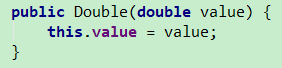Double(String s)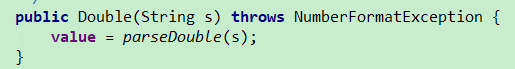常用方法

对于浮点数,有一些额外的属性方法

我们浮点数介绍中,对于浮点数的表示形式进行了介绍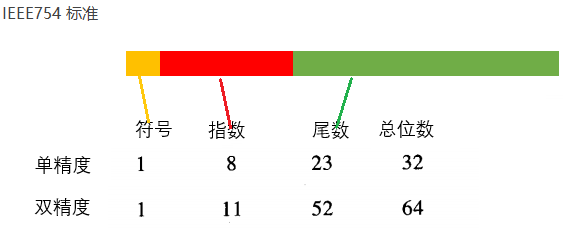Double提供了对于指定值的表示形式的获取方法, 这表示形式也就是是一个64位的二进制位序列

Double 获取表示形式
对于获取表示形式 提供了两种形式的方法,主要是针对于非数字的NaN的不同表示
他们可以与longBitsToDouble 可以进行互相转换
doubleToRawLongBits(double)

根据 IEEE 754 浮点“双精度格式”位布局，返回指定浮点值的表示形式，并保留 NaN 值

如果参数是正无穷大，则结果为 0x7ff0000000000000L

如果参数是负无穷大，则结果为 0xfff0000000000000L

如果参数是 NaN，则结果是表示实际 NaN 值的 long 整数

与 doubleToLongBits 方法不同

doubleToRawLongBits 并没有将 NaN 编码为一个“规范的”NaN 值

在所有情况下，结果都是一个 long 整数

将其赋予 longBitsToDouble(long) 方法将生成一个与 doubleToRawLongBits 的参数相同的浮点值

本地方法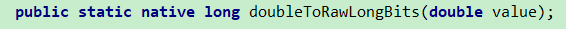doubleToLongBits(double)

根据 IEEE 754 浮点双精度格式 ("double format") 位布局，返回指定浮点值的表示形式

如果参数是正无穷大，则结果为 0x7ff0000000000000L

如果参数是负无穷大，则结果为 0xfff0000000000000L

如果参数是 NaN，则结果为 0x7ff8000000000000L

在所有情况下，结果都是一个 long 整数

将其赋予 longBitsToDouble(long) 方法将生成一个与 doubleToLongBits 的参数相同的浮点值

(所有 NaN 值被压缩成一个“规范”NaN 值时除外）

也就是NaN的处理不一样,此方法将NaN全部规范化为指定固定的值

依赖  doubleToRawLongBits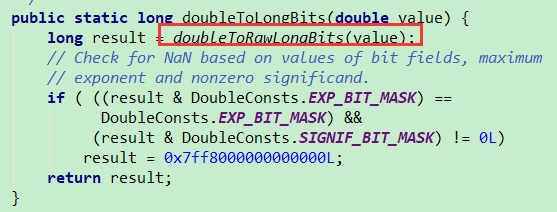longBitsToDouble(long)
返回对应于给定位表示形式的 double 值

如果参数是 0x7ff0000000000000L，则结果为正无穷大

如果参数是 0xfff0000000000000L，则结果为负无穷大

如果参数值在 0x7ff0000000000001L 到 0x7fffffffffffffffL 之间或者在 0xfff0000000000001L 到 0xffffffffffffffffL 之间，则结果为 NaN

浮点数有几种特殊的表示,比如 无穷 NaN等

额外的,也提供了一些相关的方法

static boolean isNaN(double v)
静态方法
是否一个非数字 (NaN) 值
非数值 true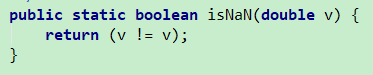static boolean isFinite(double d)
静态方法
是否是有限的浮点数
有限的true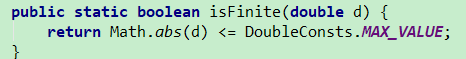static boolean isInfinite(double v)
静态方法
是否是无穷大
是无穷大  true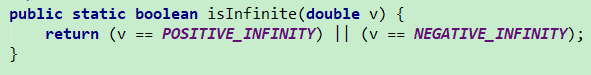boolean isInfinite()
实例方法
依赖静态方法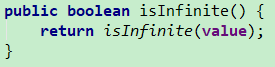boolean isNaN()

实例方法

依赖静态方法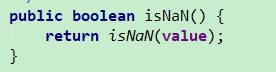比较

static int compare(double d1, double d2)
静态方法
比较两个double
d1 < d2 小于0
d1 = d2 等于0
d1 > d2 大于0
int compareTo(Double anotherDouble)
实例方法
两个对象进行大小比较,依赖于静态方法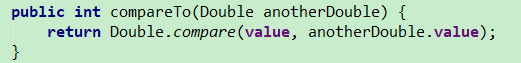parseXXX系列

字符串解析 为 基本类型,

不需要对象,所以都是静态方法返回一个字符串形式表示的基本类型double
表现效果同valueOf(String),不过valueOf 返回的是对象
如果不包含可以解析的字符串将会抛出异常
底层依赖sun.misc.FloatingDecimal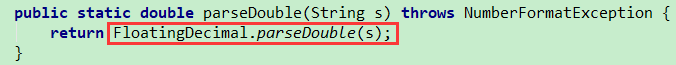valueOf系列

把基本基本类型 包装为对象

用来创建获得对象,所以无需对象,全都是静态方法不同于之前介绍的整数 数值,他们都有缓冲

Double v不存在缓存,valueOf也是直接new 对象

static Double valueOf(double d)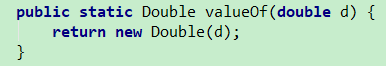static Double valueOf(String s)
依赖parseDouble方法
所以上面说跟valueOf(String)表现效果相同,本身就是一样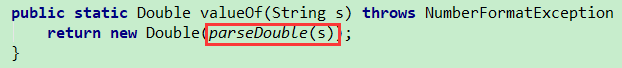Double没有 decode方法

XXXValue系列

类似之前介绍的其他数值类型
全部都是强转内部的  value
return (XXX)value;

byteValue()

shortValue()

intValue()

longValue()

floatValue()

doubleValue()

toString  toXXXString  系列

toString(double)

toString()

toHexString(double)

static String toString(double d)
静态方法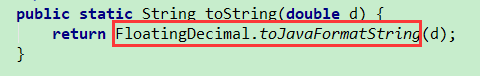String toString()
实例方法
内部调用  static String toString(double d)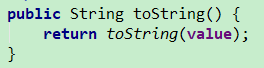static String toHexString(double d)
静态方法
返回 double参数的十六进制字符串表示形式
API帮助文档中对于字符的转换有明确的规定,可以仔细研究下
示例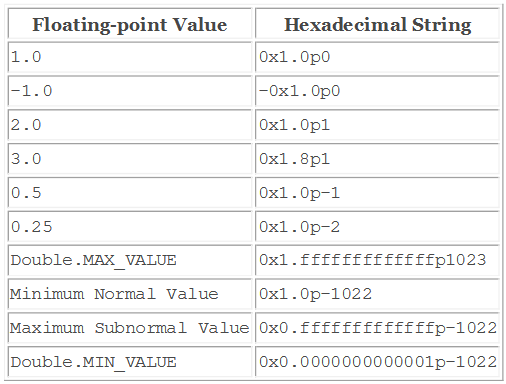toString系列好像没什么好说的,又好像有很多要说的

用到的时候对于格式字符的规定有疑惑直接查看API

equals

boolean equals(Object obj)

将此对象与指定对象比较

当且仅当参数不是 null 而是 Double 对象，且表示的 Double 值与此对象表示的 double 值相同时，结果为 true

为此，当且仅当将方法 doubleToLongBits(double) 应用于两个值所返回的 long 值相同时，才认为这两个 double 值相同

注意，在大多数情况下，对于 Double 类的两个实例 d1 和 d2，当且仅当

d1.doubleValue() == d2.doubleValue()

为 true 时，d1.equals(d2) 的值才为 true

但是，有以下两种例外情况：

如果 d1 和 d2 都表示 Double.NaN，那么即使 Double.NaN==Double.NaN 值为 false，equals 方法也将返回 true

如果 d1 表示 +0.0 而 d2 表示 -0.0，或者相反，那么即使 +0.0==-0.0 值为 true，equals 测试也将返回 false

此定义使得哈希表得以正确操作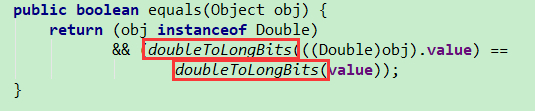hashCode

static int hashCode(double value)
静态方法
获得一个value的hashcode值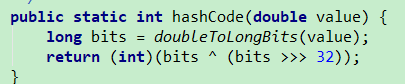int hashCode()
实例方法
依赖静态方法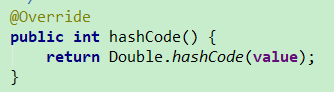其他方法

sum(double, double)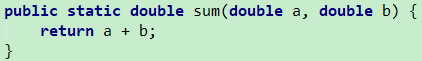max(double, double)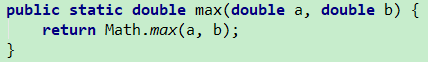min(double, double)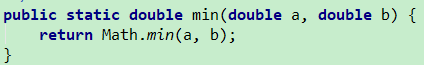总结

其实浮点数的表示形式与使用规范才是重点

就像Float似的, Double只是double的包装,double也只是IEEE754 标准的一个实现,根本还是在于标准的理解

Double  和 Float 提供的方法结构基本上是一样的,毕竟都是浮点数,标准也都是IEEE754

至此,已经介绍了,基本类型包装类中的数值部分

也就是Byte  Short  Integer Long Float Double   他们作为数值有很多类似的方法

这些类似的方法属性也可以说是作为数值类型的共性

个人认为,找到共性能够更好理解运用 java提供的类与功能展开全文• #include #include #include using namespace std; int main() { cout ************size**************"; cout 所占字节数：" (bool); cout ::max
#include<iostream>
#include<string>
#include <limits>
using namespace std;

int main()
{
cout << "type: \t\t" << "************size**************"<< endl;
cout << "bool: \t\t" << "所占字节数：" << sizeof(bool);
cout << "\t最大值：" << (numeric_limits<bool>::max)();
cout << "\t\t最小值：" << (numeric_limits<bool>::min)() << endl;
cout << "char: \t\t" << "所占字节数：" << sizeof(char);
cout << "\t最大值：" << (numeric_limits<char>::max)();
cout << "\t\t最小值：" << (numeric_limits<char>::min)() << endl;
cout << "signed char: \t" << "所占字节数：" << sizeof(signed char);
cout << "\t最大值：" << (numeric_limits<signed char>::max)();
cout << "\t\t最小值：" << (numeric_limits<signed char>::min)() << endl;
cout << "unsigned char: \t" << "所占字节数：" << sizeof(unsigned char);
cout << "\t最大值：" << (numeric_limits<unsigned char>::max)();
cout << "\t\t最小值：" << (numeric_limits<unsigned char>::min)() << endl;
cout << "wchar_t: \t" << "所占字节数：" << sizeof(wchar_t);
cout << "\t最大值：" << (numeric_limits<wchar_t>::max)();
cout << "\t\t最小值：" << (numeric_limits<wchar_t>::min)() << endl;
cout << "short: \t\t" << "所占字节数：" << sizeof(short);
cout << "\t最大值：" << (numeric_limits<short>::max)();
cout << "\t\t最小值：" << (numeric_limits<short>::min)() << endl;
cout << "int: \t\t" << "所占字节数：" << sizeof(int);
cout << "\t最大值：" << (numeric_limits<int>::max)();
cout << "\t最小值：" << (numeric_limits<int>::min)() << endl;
cout << "unsigned: \t" << "所占字节数：" << sizeof(unsigned);
cout << "\t最大值：" << (numeric_limits<unsigned>::max)();
cout << "\t最小值：" << (numeric_limits<unsigned>::min)() << endl;
cout << "long: \t\t" << "所占字节数：" << sizeof(long);
cout << "\t最大值：" << (numeric_limits<long>::max)();
cout << "\t最小值：" << (numeric_limits<long>::min)() << endl;
cout << "unsigned long: \t" << "所占字节数：" << sizeof(unsigned long);
cout << "\t最大值：" << (numeric_limits<unsigned long>::max)();
cout << "\t最小值：" << (numeric_limits<unsigned long>::min)() << endl;
cout << "double: \t" << "所占字节数：" << sizeof(double);
cout << "\t最大值：" << (numeric_limits<double>::max)();
cout << "\t最小值：" << (numeric_limits<double>::min)() << endl;
cout << "long double: \t" << "所占字节数：" << sizeof(long double);
cout << "\t最大值：" << (numeric_limits<long double>::max)();
cout << "\t最小值：" << (numeric_limits<long double>::min)() << endl;
cout << "float: \t\t" << "所占字节数：" << sizeof(float);
cout << "\t最大值：" << (numeric_limits<float>::max)();
cout << "\t最小值：" << (numeric_limits<float>::min)() << endl;
cout << "size_t: \t" << "所占字节数：" << sizeof(size_t);
cout << "\t最大值：" << (numeric_limits<size_t>::max)();
cout << "\t最小值：" << (numeric_limits<size_t>::min)() << endl;
cout << "string: \t" << "所占字节数：" << sizeof(string) << endl;
// << "\t最大值：" << (numeric_limits<string>::max)() << "\t最小值：" << (numeric_limits<string>::min)() << endl;
cout << "type: \t\t" << "************size**************"<< endl;
return 0;
}/*运行结果分析：
以上结果已经很明白了，一下补充说明几点：
概念、整型：表示整数、字符和布尔值的算术类型合称为整型（integral type）。
关于带符号与无符号类型：整型 int、stort  和  long 都默认为带符号型。要获得无符号型则必须制定该类型为unsigned，比如unsigned long。unsigned int类型可以简写为unsigned，也就是说，unsigned后不加其他类型说明符就意味着是unsigned int。
一字节表示八位，即：1byte = 8 bit;
int: 4byte =  32 bit 有符号signed范围：2^31-1 ~ -2^31即：2147483647 ~ -2147483648无符号unsigned范围：2^32-1 ~ 0即：4294967295
~ 0
long: 4 byte = 32 bit 同int型
double: 8 byte = 64 bit 范围：1.79769e+308 ~ 2.22507e-308
long double: 12 byte = 96 bit 范围： 1.18973e+4932 ~ 3.3621e-4932
float: 4 byte = 32 bit 范围： 3.40282e+038 ~ 1.17549e-038
int、unsigned、long、unsigned long 、double的数量级最大都只能表示为10亿，即它们表示十进制的位数不超过10个，即可以保存所有9位整数。而short只是能表示5位；

另外对于浮点说而言：使用double类型基本上不会有错。在float类型中隐式的精度损失是不能忽视的，二双精度计算的代价相对于单精度可以忽略。事实上，在有些机器上，double类型比float类型的计算要快得多。float型只能保证6位有效数字，而double型至少可以保证15位有效数字（小数点后的数位），long double型提供的精度通常没有必要，而且还要承担额外的运行代价。
double是8字节共64位，其中小数位占52位，2-^52=2.2204460492503130808472633361816e-16，量级为10^-16，故能够保证2^-15的所有精度。
在有些机器上，用long类型进行计算所付出的运行时代价远远高于用int类型进行同样计算的代价，所以算则类型前要先了解程序的细节并且比较long类型与int类型的实际运行时性能代价。

欢迎提出宝贵意见，以帮助我改进，不胜感激！！！
——桑海整理


展开全文•  float和double的范围是由指数的位数来决定的。  float的指数位有8位，而double的指数位有11位，分布如下：  float：  1bit（符号位） 8bits（指数位） 23bits（尾数位）  double： 1bit（符号位） 11bits...
1. 范围
float和double的范围是由指数的位数来决定的。
float的指数位有8位，而double的指数位有11位，分布如下：
float：
1bit（符号位） 8bits（指数位） 23bits（尾数位）
double：  1bit（符号位） 11bits（指数位） 52bits（尾数位）
于是，float的指数范围为-127~+128，而double的指数范围为-1023~+1024，并且指数位是按补码的形式来划分的。
其中负指数决定了浮点数所能表达的绝对值最小的非零数；而正指数决定了浮点数所能表达的绝对值最大的数，也即决定了浮点数的取值范围。
float的范围为-2^128 ~ +2^128，也即-3.40E+38 ~ +3.40E+38；double的范围为-2^1024 ~ +2^1024，也即-1.79E+308 ~ +1.79E+308。

2.  精度  float和double的精度是由尾数的位数来决定的。浮点数在内存中是按科学计数法来存储的，其整数部分始终是一个隐含着的“1”，由于它是不变的，故不能对精度造成影响。
float：2^23 = 8388608，一共七位，这意味着最多能有7位有效数字，但绝对能保证的为6位，也即float的精度为6~7位有效数字；
double：2^52 = 4503599627370496，一共16位，同理，double的精度为15~16位。（能绝对保证15位没有问题）

3.Oracle中Number类型

在Oracle中Number类型可以用来存储0，正负定点或者浮点数，可表示的数据范围在
1.0 * 10(-130) —— 9.9...9 * 10(125) {38个9后边带88个0}
的数字，当Oracle中的数学表达式的值>=1.0*10(126)时，Oracle就会报错。
Number的数据声明如下：
表示        作用        说明
Number(p, s)        声明一个定点数        p(precision)为精度，s(scale)表示小数点右边的数字个数，精度最大值为38，
Number(p)        声明一个整数        相当于Number(p, 0)
Number        声明一个浮点数        其精度为38，要注意的是scale的值没有应用，也就是说scale的指不能简单的理解为0，或者其他的数。

定点数的精度(p)和刻度(s)遵循以下规则：
?        当一个数的整数部分的长度 > p-s 时，Oracle就会报错
?        当一个数的小数部分的长度 > s 时，Oracle就会舍入。
?        当s(scale)为负数时，Oracle就对小数点左边的s个数字进行舍入。
?        当s > p 时, p表示小数点后第s位向左最多可以有多少位数字，如果大于p则Oracle报错，小数点后s位向右的数字被舍入

4.验证
create or replace function  func_test(p_type number) return number
is
/*
功能：基于警度图数据同步
*/
l_cnt number;
begin
select p_type into l_cnt from dual;
return l_cnt;
end func_test;
/
show err;

5.结论

number 的总长度是40位，其中可能包括：小数点，负号位。

select to_char(func_test(-987.1234567891234567891234567891234567891234)) from dual;
-987.12345678912345678912345678912345679   //包括小数点及负号位共40位
select to_char(func_test(9876.1234567891234567891234567891234567891234)) from dual;
9876.12345678912345678912345678912345679   //4位整数+小数点+35位小数=40位
select to_char(func_test(987.1234567891234567891234567891234567891234)) from dual;
987.123456789123456789123456789123456789   //3位整数+小数点+36位小数=40位
select to_char(func_test(1234567891234567891234567891234567891234)) from dual;
1234567891234567891234567891234567891234   //40位整数
select to_char(func_test(12345678912345678912345678912345678912345)) from dual;
1.2345678912345678912345678912345679E+40   //41位时精度发生丢失
1.2345678912345678912345678912345679×10^40 即 12345678912345678912345678912345678900000
展开全文• ## double和Double的区别

万次阅读 多人点赞 2017-08-21 17:43:46
double是基本数据类型，Double是原始数据类型 double没有方法，Double有自己的属性和方法 double只创建引用，Double创建对象 集合类不能存放double，只能存放Double double存放在栈中，Double存放在堆中 栈的...
double是基本数据类型，Double是原始数据类型double没有方法，Double有自己的属性和方法double只创建引用，Double创建对象集合类不能存放double，只能存放Doubledouble存放在栈中，Double存放在堆中栈的存取速度要高于堆，另外栈中的数据可以共享如：double a = 0;double b = 0;不会创建对象，只会建立两个引用，同时指向变量“0”（栈数据共享）而Double a = new Double(0);Double b = new Double(0);会创建两个对象，即使对象所代表的值一样（堆数据不共享）所以从效率来讲用double合适，而Double提供的属性和方法会使操作各种操作更加方便和灵活

展开全文• ## Double

千次阅读 2018-04-11 00:54:04
Dubbo功能介绍 Dubbo的发展史 Dubbo是阿里巴巴内部的SOA服务化治理方案的核心框架，每天为2000+ 个服务提供3,000,000,000+ 次访问量支持，并被广泛应用于阿里巴巴集团的各成员站点。Dubbo自2011年开源后，已被...
• 为什么80%的码农都做不了架构师？>>> ...
• 在Java中double的运算是不精确的，比如doule a=0.1;double b=0.2，但是a+b!=0.3 由于Java的简单类型不能够精确的对浮点数进行运算，这个工具类Arith提供精确的浮点数运算，包括加减乘除和四舍五入。但是需要注意...
• Double 类在对象中包装了一个基本类型 double 的值。Double 类对象包含一个 double 类型的字段。此外，该类还提供了多个方法，可以将 double 类型与 String 类型相互转换，同时 还提供了处理 double 类型时比较常用...Java Java类
• doubleDouble double是基本数据类型，Double是原始数据类型 double没有方法，Double有自己的属性和方法 double只创建引用，Double创建对象 集合类不能存放double，只能存放Double double存放在栈中，Double存放在...
• 这里写自定义目录标题把double[]存入Json的准备工作List<> ,double[ ], Double[ ]数据类型特性分析List转double[ ]double[ ] 转List 把double[]存入Json的准备工作 最近想将double[] 用json格式保存，发现json...Json List 基本数据类型
• 1、Double是java定义的类，而double是预定义数据类型（8种中的一种） 2、Double就好比是对double类型的封装，内置很多方法可以实现String到double的转换，以及获取各种double类型的属性值（MAX_VALUE、SIZE等等） ...Java面试题
• ## 深入理解双指针

万次阅读 热门讨论 2010-02-09 15:38:00
深入理解双指针 对于C语言的参数传递都是值传递，当传传递一个指针给函数的时，其实质上还是值传递，除非使用双指针。 在讲双指针之前，还是先讲讲关于C语言函数调用的本质。函 数调用操作包括从一块代码到另一块...list 语言 汇编 struct null
• public class test09 { public static void main(String[] args) { double a = 5000.44; double b = 100.12; double v = a / b; int i = new Double(v).intValue(); ...double转int
• ## c语言double类型printf问题

万次阅读 多人点赞 2018-10-03 11:32:10
结论：在c语言中，double类型的读入必须用"%lf"；输出必须用"%f" 解释：double和float的精度是不同的，故存储空间也是不同的，所以如果要读入double类型，必须要用%lf来读入，以免精度丢失。而输出，由于...c printf
• ## JAVA double类型相加

万次阅读 2012-06-16 17:24:44
问题的提出： 编译运行下面这个程序会看到什么？ public class Test{  public static void main(String args[]){  System.out.println(0.05+0.01);  System.out.prjava string integer div
• 转载自：Java中的Double类型计算 一、问题的提出： 如果我们编译运行下面这个程序会看到什么？ public class Test{  public static void main(String args[]){  System.out.println(0.05+0.01)...java
• 调用方法Double.parseDouble 注意Double 的 D 是大写的！c++ coutc++
• =0.9的问题，这主要和double的精度有关，涉及到double在计算机内的存储结构的问题，这个可以参考这篇博客：http://blog.csdn.net/softwater007/article/details/3330619，另外double类型的存储符合IEEE754标准，相关...
• 在Java中int类型数据的大小比较可以使用双等号，double类型则不能使用双等号来比较大小，如果使用的话得到的结果将永远是不相等，即使两者的精度是相同的也不可以。下面介绍两种比较double数据是否相等的方法。第一...
• ## Double类型精度问题

千次阅读 2018-08-08 17:06:27
public static void main(String[] args) { double d1 = 1.1D; double d2 = 2.2D; double d3 = d1+d2; System.out.println("d1+d2= "+d3); BigDecimal b1 = new BigDecima...BigDecimal
• package util;import java.math.BigDecimal;... * 工具类 - 运算 double类型的数字相加不丢失精度 * */public class ArithUtil { // 默认除法运算精度 private static final int DEF_DIV_SCAL
• ## double类型解析

千次阅读 2016-12-08 22:58:36
double类型存储规定：把浮点数化成小数点前只有1位的小数与2的指数的乘积的形式，存储小数点后的52位，2的指数及数的符号。sizeof(double) // 8个字节#include //利用共用体的特点，所有类型共用一段最大的内存空间...
• ## double类型数据运算

千次阅读 2018-02-27 15:11:52
import java.math.BigDecimal; public class Arith { private static final int DEF_DIV_... /** * * 两个Double数相加 * * * @param v1 * * @param v2 * * @return Double */ public...
• 多个double类型的数直接相加的时候，可能存在精度误差.（ 由于计算机算法以及硬件环境决定只能识别 0 1。计算机默认的计算结果在都在一个指定精度范围之内，想往深的了解，可以学习数值分析等） 在金融方面是绝对不...java
• 下面我推荐这种方式： public static void main(String[] args) { double test1=10.2234; double test2=10.3356;... Double dtest1 = Double.valueOf(String.format("%.2f", test1 )); ...java
• /* * 如果是小数，保留两位，非小数，保留整数 * @param number ... public static String getDoubleString(double number) { String numberStr; if (((int) number * 1000) == (int) (number ...
• public static void main(String[] args) { double db = 15.87; int in = new Double(db).intValue(); System.out.println(in); } 运行结果： 15int
• BigDecimal 处理Double类型的基本运算 BigDecimal 处理Double类型的基本运算 Java Double 数据在进行数据计算的时候，很容出现丢失精度的问题，因此借助于BigDecimal进行运算，可以很好的解决这个问题。package ...Java BigDecimal 数据...